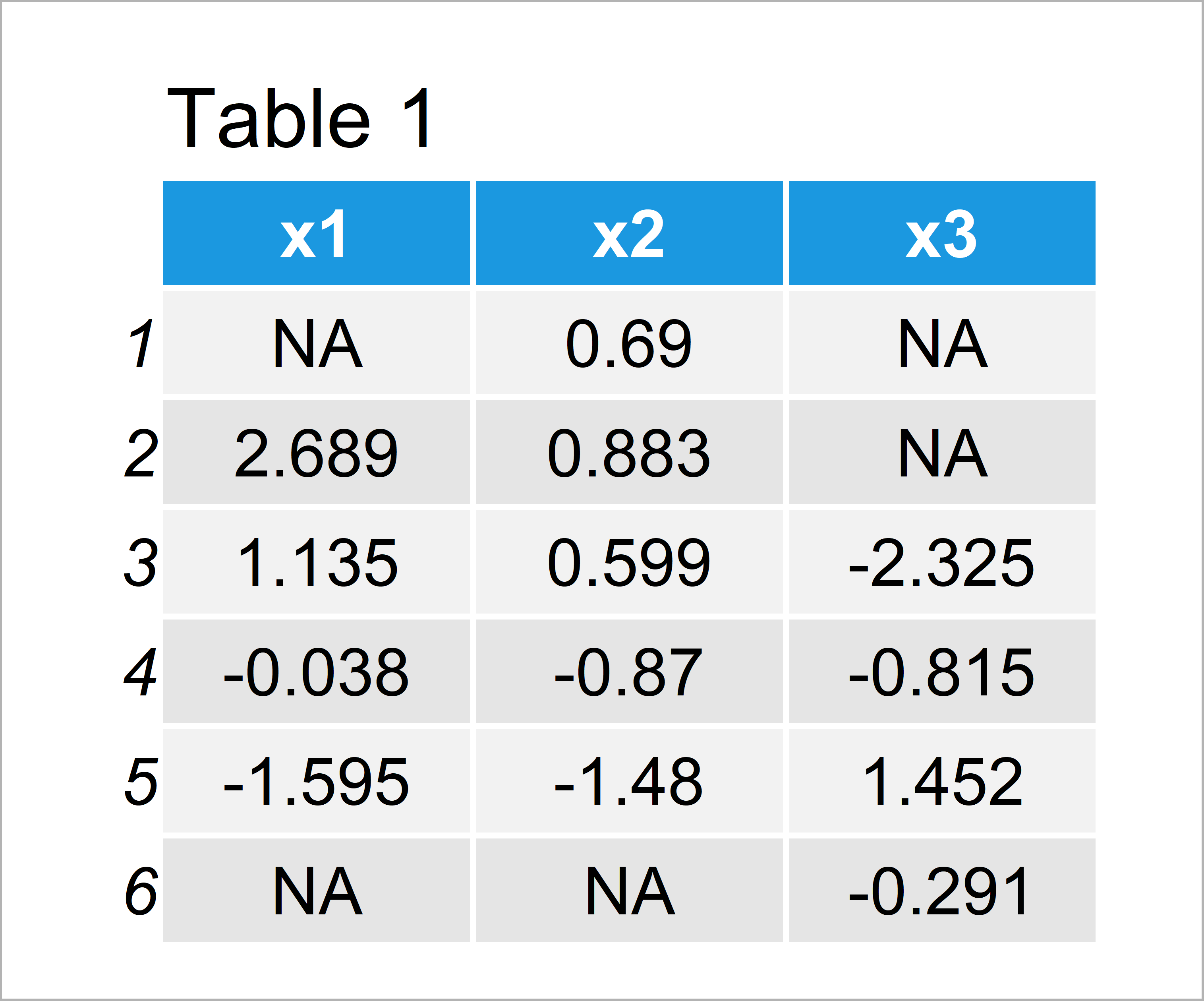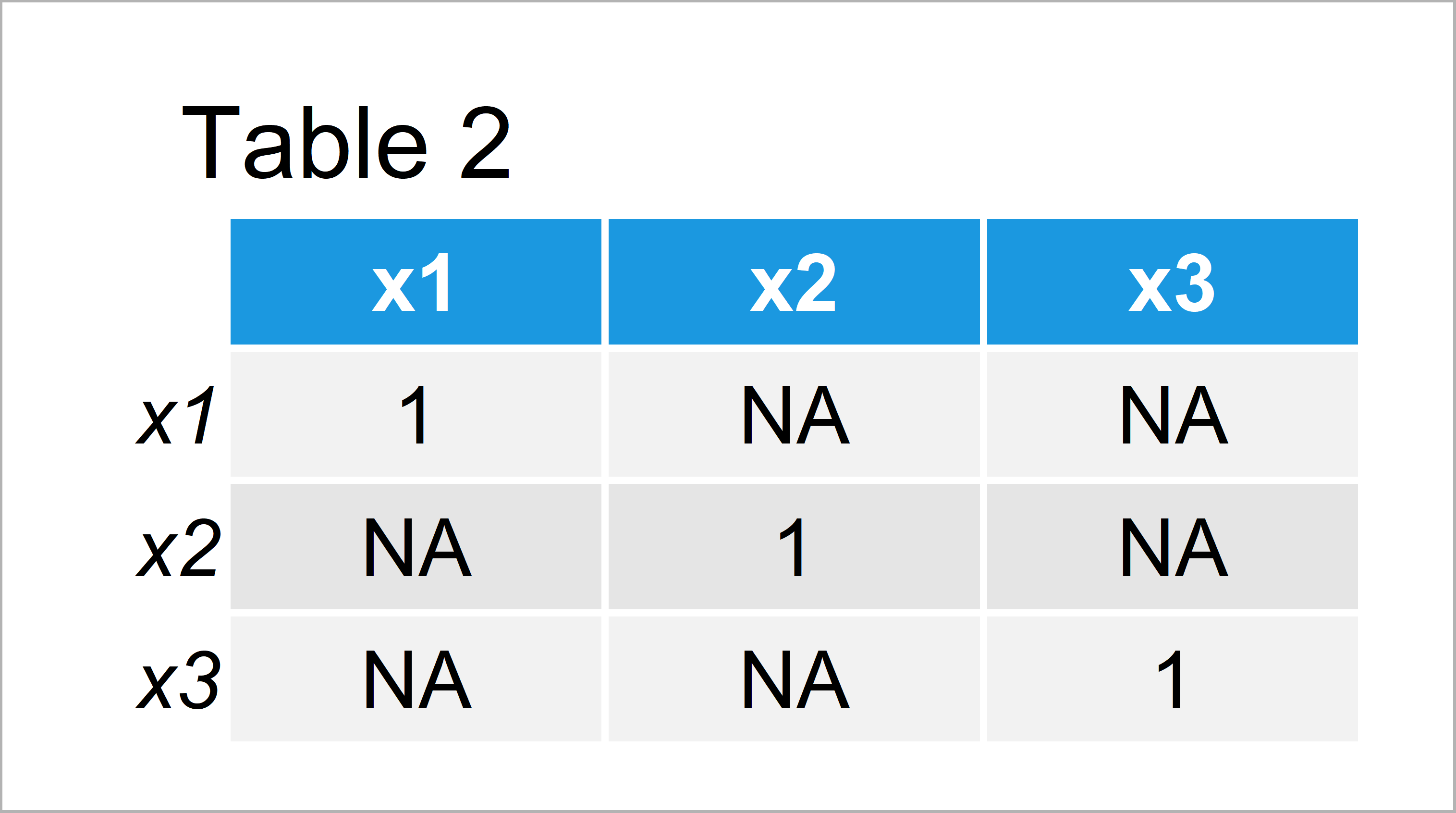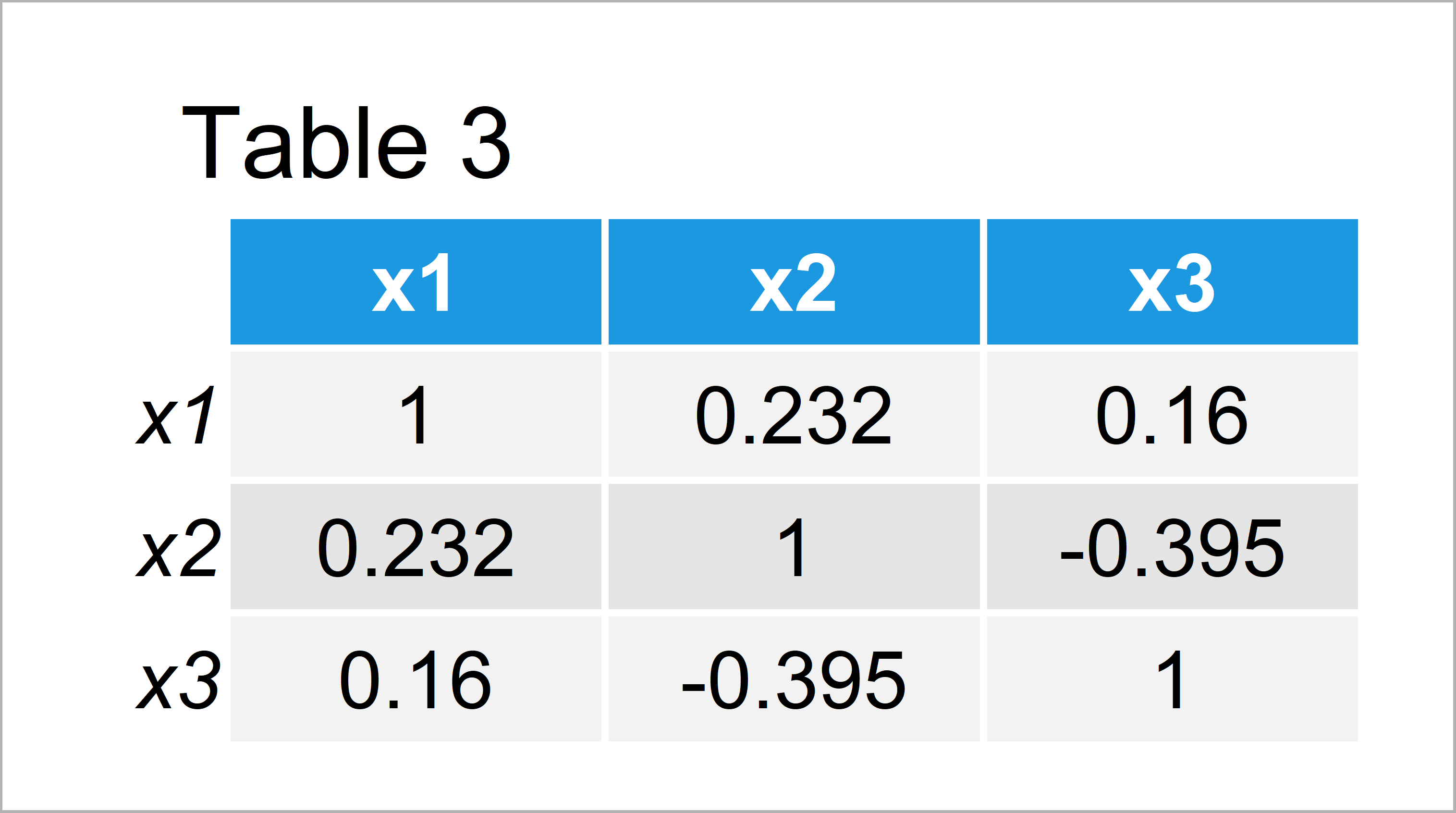# R cor Function Shows Only NA & 1 (2 Examples)

In this R programming tutorial you’ll learn how to solve the problem that a correlation matrix shows only NA and 1.

The post will consist of these content blocks:

Let’s start right away:

## Creation of Example Data

The first step is to construct some data that we can use in the following examples:

```set.seed(395176)                   # Create example data frame
x1 <- rnorm(100)
x2 <- rnorm(100) + 0.3 * x1
x3 <- rnorm(100) + 0.5 * x1 - 0.7 * x2
x1[rbinom(100, 1, 0.2) == 1] <- NA
x2[rbinom(100, 1, 0.2) == 1] <- NA
x3[rbinom(100, 1, 0.2) == 1] <- NA
data <- data.frame(x1, x2, x3)Table 1 illustrates the structure of the example data: It is made of 100 lines and three numeric columns. As you can see, some data cells of our data frame are missing.

## Example 1: Correlation Matrix with Missing Values

In Example 1, I’ll show how to reproduce the falsely specified correlation matrix that shows only NA (i.e. Not Available or missing values) and 1.

Let’s assume that we want to create a correlation matrix using the cor function:

`cor(data)                          # Apply cor() function`In Table 2 it is shown that we have created a matrix showing only NA and 1 using the previous syntax.

The reason for this is that our input data frame contains missing values.

So how can we solve this problem? That’s what I’ll explain next!

## Example 2: Remove Missing Values from Correlation Matrix

This example illustrates how to create a correlation matrix with proper correlation coefficients even though our input data contains missing values.

For this, we can set the use argument of the cor function to be equal to “complete.obs”:

`cor(data, use = "complete.obs")    # cor() function & use argument`The output of the previous R programming code is shown in Table 3 – A correlation matrix showing correlation coefficients for our input data frame.

Please note that the specification use = “complete.obs” performs listwise deletion. Make sure to check out this tutorial to learn more about the pros and cons of this method, because in some cases listwise deletion can lead to bias in your results.

## Video, Further Resources & Summary

In case you need further explanations on the R code of this article, I recommend having a look at the following video of my YouTube channel. In the video, I’m explaining the content of this tutorial.

Furthermore, you might read the related articles of my website. I have released several related tutorials already:

To summarize: In this tutorial, I have explained how to show correlation values instead of NA when using the cor() function in R. Please let me know in the comments, if you have any additional questions.

Subscribe to the Statistics Globe Newsletter